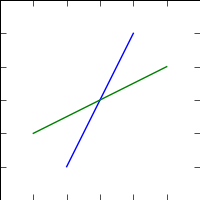# figure: centering data and plot.svg borders

Hi everyone,

Is there a straightforward way for me to ensure that my data is

centered: allways present on the figure? I’m plotting the results

from complicated geometrical calculations and I need high dpi

so that I can debug the code. I’ve recorded the session in a log

file and I’ve modified the ipython logfile into a script. The

result is here:

http://img87.imageshack.us/img87/8306/interfaceprojections.png

and the script is here:

http://pastebin.com/3dkfNDuk

As you see, in the image, a part of the rectangle is missing. It is

actually sort of a rectangle shaped set of finite volumes. What

I’m looking for is to have the whole rectangle (or any other shape)

visible on the axes of the figure, with a properly scaled axes ratio

(‘equal’ in the script). And to reduce the output, could it be possible

to plot just the diagram, without the remaining whitespace?

It seems that when I use

figureOne.set_figheight(number)

figureOne.set_figwidth(number)

it stands for the figure, but not for the axes. I’m interested just in

the axes since it’s the only subplot.

I looked in the user guide but I didn’t find anything so far… I’ll keep

looking.

Tomislav

Maybe:

from matplotlib import pyplot as plt

figureOne = plt.figure()
axesOne = figureOne.add_axes([0, 0, 1, 1])
axesOne.plot([-1, 1], [-2, 2])
axesOne.plot([-2, 2], [-1, 1])

axesOne.set_xlim((-3, 3))
axesOne.set_ylim((-3, 3))
figureOne.set_figwidth(2)
figureOne.set_figheight(2)

plt.savefig("Friedrich.png")

Friedrich